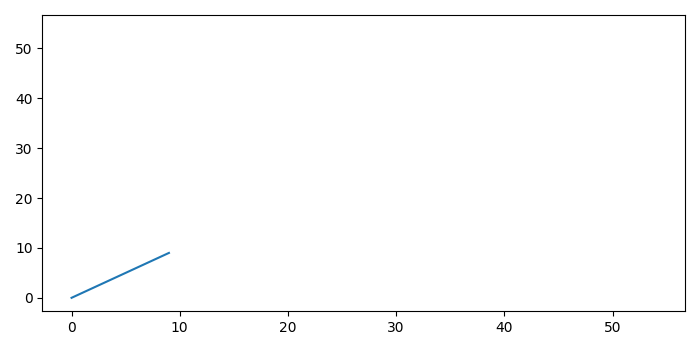# Automatically Rescale ylim and xlim in Matplotlib

MatplotlibServer Side ProgrammingProgramming

#### Python Data Science basics with Numpy, Pandas and Matplotlib

Most Popular

63 Lectures 6 hours

#### Data Visualization using MatPlotLib & Seaborn

11 Lectures 4 hours

#### MatPlotLib with Python

9 Lectures 2.5 hours

To rescale ylim and xlim automatically, we can take the following steps −

• To plot a line, use plot() method and data range from 0 to 10.

• To scale the xlim and ylim automatically, we can make the variable scale_factore=6.

• Use scale_factor (from Step 2) to rescale the xlim and ylim, using xlim() and ylim() methods, respectively.

• To display the figure, use show() method.

## Example

from matplotlib import pyplot as plt
plt.rcParams["figure.figsize"] = [7.00, 3.50]
plt.rcParams["figure.autolayout"] = True
plt.plot(range(0, 10))
scale_factor = 6
xmin, xmax = plt.xlim()
ymin, ymax = plt.ylim()
plt.xlim(xmin * scale_factor, xmax * scale_factor)
plt.ylim(ymin * scale_factor, ymax * scale_factor)
plt.show()

## Output